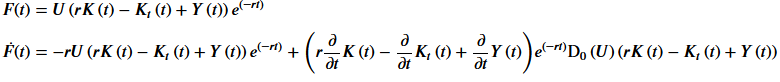# One more time : two problems of substitutionAs there is no module about calculus of variations (or optimal control), I try to write the Euler equation from scratch

t, T, K_0, K_T, r=var('t T K_0 K_T r')
assume(r>0)
assume(K_0>0)
assume(K_T>0)
U=function('U')
Y=function('Y')
K=function('K')
K_t=function('K_t')
F=function('F')(t)
F(t)=e^(-r*t)*U(H(t))
F_t=function('F_t')
U_t=function("U'")(x)
F_t= diff(F,t)
H=function('H')
H(t) = r*K(t)-K_t(t)+Y(t)
show(LatexExpr(r'F(t) = '),F(t))
show(LatexExpr(r'\dot{F}(t) = '),F_t(t))


which givesHere I encounter two difficulties :

1) I want substitute to $D_0(U)$ the standard notation for univariate derivative (that is $U^\prime()$). But, dispite all the good advices which are in the answer to my question https://ask.sagemath.org/question/782... I cannot obtain any result.

2) I would like to substitute later to the unformall $U(x)$ the function $x^a$ (or any other admissible functon like $U(x) = \log(x)$). Also I must declare a function fo $Y(t)$.This time also I cannot obtain what I am searching for.

I am sincerely sorry to be so uneffective in fiding some solutions.

edit retag close merge delete

Sort by » oldest newest most voted

First : as given, your code does not run, some functions being used before definition. Please do your homework and check your sample code after reset()ting before posting.

Your problem is that the operator of a derivative expression is not recognized as a function by substitute_function. After running :

t, T, K_0, K_T, r=var('t T K_0 K_T r')
assume(r>0)
assume(K_0>0)
assume(K_T>0)
U=function('U')
Y=function('Y')
K=function('K')
K_t=function('K_t')
# H=function('H') # Superfluous, ,since H is defined immediately below
H(t) = r*K(t)-K_t(t)+Y(t) # H must be defined fefore being used in F(t)
# F=function('F')(t) # Superfluous, ,since F is defined immediately below
F(t)=e^(-r*t)*U(H(t))
# F_t=function('F_t') # Superfluous, since f_t is defined two lines below.
# U_t=function("U'")(x)
U_t=function("U'")
F_t= diff(F,t)


view(LatexExpr("F(t)=%s"%latex(F(t))))


which displays : $$\dot{F}(t)=U\left(r K\left(t\right) - K_{t}\left(t\right) + Y\left(t\right)\right) e^{\left(-r t\right)}$$

The expression to be reexpressed is

sage: foo=F_t(t).maxima_methods().part(1,1) ; foo
D(U)(r*K(t) - K_t(t) + Y(t))


(This site's Mathjax isn't able to typeset this, bur LeTeX can).

Whose operator (= function) is

sage: foo.operator()
D(U)


We should be able to write :

     F_t().substitute_function(F_t(t).maxima_methods().part(1,1).operator(), U_t)


But this does not work, this operator being not recognized as a function :

sage: F_t().substitute_function(F_t(t).maxima_methods().part(1,1).operator(), U_t)
---------------------------------------------------------------------------
TypeError                                 Traceback (most recent call last)
Cell In , line 1
----> 1 F_t().substitute_function(F_t(t).maxima_methods().part(Integer(1),Integer(1)).operator(), U_t)

File /usr/local/sage-10/src/sage/symbolic/expression.pyx:6063, in sage.symbolic.expression.Expression.substitute_function()
6061 else:
6062     for a in args:
-> 6063         _dict_update_check_duplicate(sdict, _subs_fun_make_dict(a))
6064
6065 if kwds:

File /usr/local/sage-10/src/sage/symbolic/expression.pyx:724, in sage.symbolic.expression._subs_fun_make_dict()
722 else:
723     msg = "not able to determine a substitution from {}"
--> 724     raise TypeError(msg.format(s))
725
726

TypeError: not able to determine a substitution from D(U)


The key is that :

sage: foo.operator() in SR
False


Complain fiercely. Or file a well-documented issue...

The workaround is to replace the whole subexpression foo in the expression you want to reformat, which gives :

view(LatexExpr(r"\dot{F}(t)=%s"%latex(F_t(t).subs({foo:U_t(*foo.operands())}))))


$$\dot{F}(t)=-r U\left(r K\left(t\right) - K_{t}\left(t\right) + Y\left(t\right)\right) e^{\left(-r t\right)} + {\left(r \frac{\partial}{\partial t}K\left(t\right) - \frac{\partial}{\partial t}K_{t}\left(t\right) + \frac{\partial}{\partial t}Y\left(t\right)\right)} {\rm U'}\left(r K\left(t\right) - K_{t}\left(t\right) + Y\left(t\right)\right) e^{\left(-r t\right)}$$

For your second qyuuestion the substitution has no role, because you can substitute U before differentiating ; no further derivative ioerators in sight :

sage: F(t).substitute_function(U, (x^a).function(x)).diff(t)
(r*diff(K(t), t) - diff(K_t(t), t) + diff(Y(t), t))*(r*K(t) - K_t(t) + Y(t))^(a - 1)*a*e^(-r*t) - (r*K(t) - K_t(t) + Y(t))^a*r*e^(-r*t)


$$-r e^{\left(-r t\right)} \log\left(r K\left(t\right) - K_{t}\left(t\right) + Y\left(t\right)\right) + \frac{{\left(r \frac{\partial}{\partial t}K\left(t\right) - \frac{\partial}{\partial t}K_{t}\left(t\right) + \frac{\partial}{\partial t}Y\left(t\right)\right)} e^{\left(-r t\right)}}{r K\left(t\right) - K_{t}\left(t\right) + Y\left(t\right)}$$

sage: a=SR.var("a")
sage: F(t).substitute_function(U, (x^a).function(x)).diff(t)
(r*diff(K(t), t) - diff(K_t(t), t) + diff(Y(t), t))*(r*K(t) - K_t(t) + Y(t))^(a - 1)*a*e^(-r*t) - (r*K(t) - K_t(t) + Y(t))^a*r*e^(-r*t)


$${\left(r \frac{\partial}{\partial t}K\left(t\right) - \frac{\partial}{\partial t}K_{t}\left(t\right) + \frac{\partial}{\partial t}Y\left(t\right)\right)} {\left(r K\left(t\right) - K_{t}\left(t\right) + Y\left(t\right)\right)}^{a - 1} a e^{\left(-r t\right)} - {\left(r K\left(t\right) - K_{t}\left(t\right) + Y\left(t\right)\right)}^{a} r e^{\left(-r t\right)}$$

HTH,

more